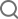Search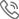+86-0755-28772795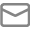sales@halnziye.com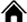> Home>Contact Us>Latest News> Thermal Conductivity Testing Methods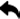Return to List

Thermal Conductivity Testing Methods

Thermal conductivity measurements can be divided into two groups: steady state and transient state testing methods. Steady state methods also includes guarded hot-plate (GHP) method and Heat-Flow Meter (HFM), while a typical transient method is Laser/Xenon Flash (LFA/XFA) method.

GUARDED HOT-PLATE (GHP) METHOD (ASTM C177)

The figure below is the schematic view of the setup for GHP method. Thermal conductivity can be calculated by the following equation, where k is thermal conductivity, Q/t is the heat flow rate, A is the area of the samples, delta x is thickness, delta T is the temperature gradience. More detailed description about this method can be found in this linked publication. The above figure comes from Computers and Concrete · September 2016.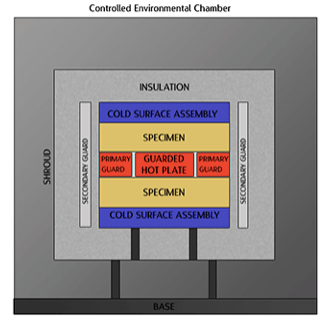https://thermtest.com/history-2-the-guarded-hot-plate-method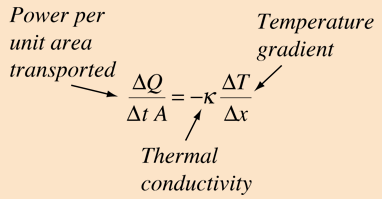from hyper physics

HEAT-FLOW METER (HFM) MEHTOD (ASTM C518 AND ASTM E1530)

The heat flow method uses two calibrated heat flux transducers to measure heat flux through a specimen that is placed between them as shown in the following figure. A steady-state unidirectional heat flux is maintained by applying a given thermal gradient across the specimen. Thermal conductivity can be calculated by same equation as in the GHP method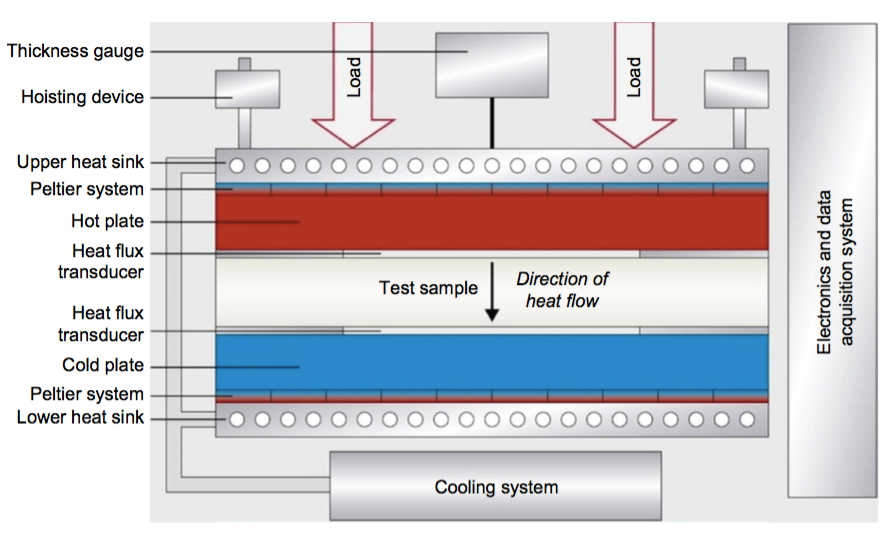The challenge of this steady state technology is to minimize parasitic heat losses to a very low level and overcome the influences of contact resistance between sample an metal bars. The steady-state method from ASTM C177 and ISO8302 is adapted for thermal interface materials.

Note: ASTM E1530 method is similar in concept to Test Method C518, but is modified to accommodate smaller test specimens, having a higher thermal conductance.

HEAT-FLOW METER MEHTOD (ASTM D5470)

The steady state method is commonly adopted to measure thermal resistance and conductivity of thermal interface materials or TIMs. A heat flow through the TIM is generated and the temperature gradient across the TIM is measured, and the thermal resistance or conductivity can be calculated through the procedure defined by ASTM standard ASTM D5470.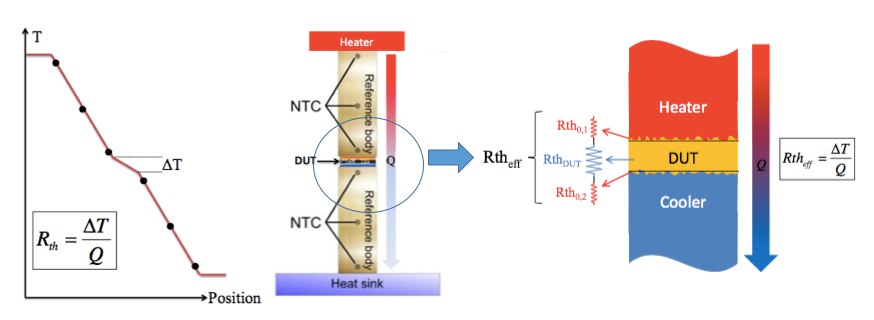The above figure schematically shows the setup and measuring principle for thermal resistance. NTC: Negative Temperature Coefficient Thermistors; DUT: Device under test, Rth: thermal resistance. Effective thermal resistance for a TIM is the sum of bulk resistance and interfacial resistance.

LASER/XENON FLASH (LFA/XFA) METHOD (ASTM E1461)

The laser or xenon flash technique is popular to determine materials’ thermal diffusivity and thermal conductivity calculated through thermal diffusivity, heat capacity, and density.

A flash of a laser or a xenon light is irradiated on one surface of a small disk-shaped sample; sample absorb the photon energy can convert to to heat, which will transfer to the other side of the sample. The resulted temperature rise Vs time is detected by a thermocouple or a pyrometer. An example of monitored temperature history is shown in the following figure, where the characteristic time,t1/2, of a 50 % rise is recorded.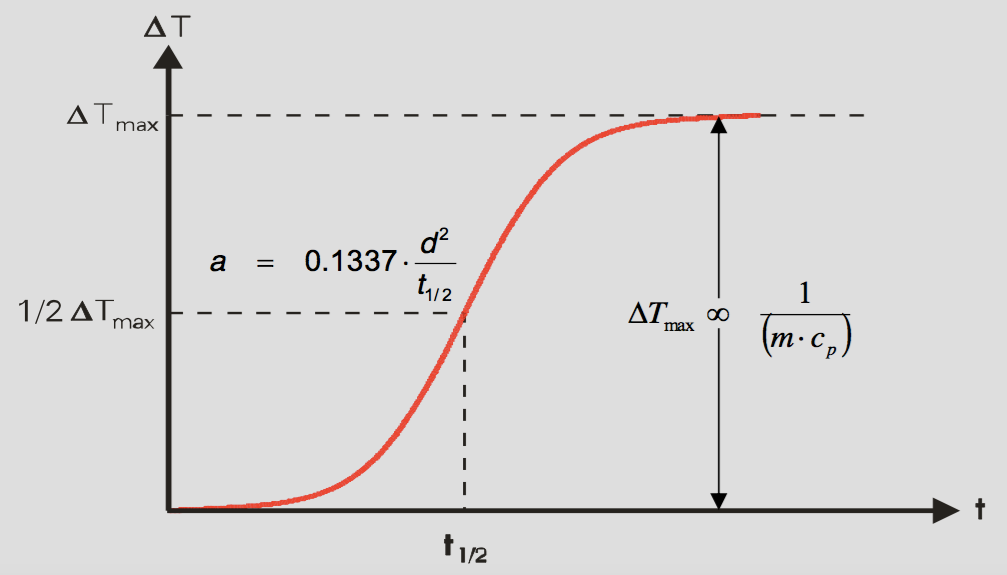Figure from Netzsch

Thermal diffusivity is calculated by the following equation, L is the sample thickness (sources: DOI 10.1007/s10765-015-1863-x).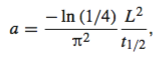By measuring material’s heat capacity and density, thermal conductivity can be calculated by the following equation: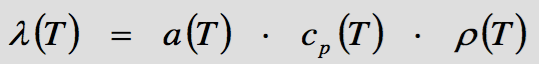In-plane mode can also determine materials’ thermal conductivity in X-Y surface. The following is TA’s setup for in-plane thermal conductivity testing.

Netzsch’s method has the same concept; only the heat transfer direction is opposite to TA’s.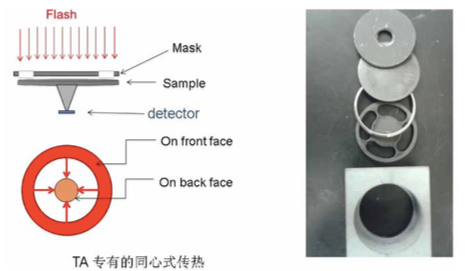The measurement and calculation of thermal conductivity is similar.×
• Products

• Solutions

• Support